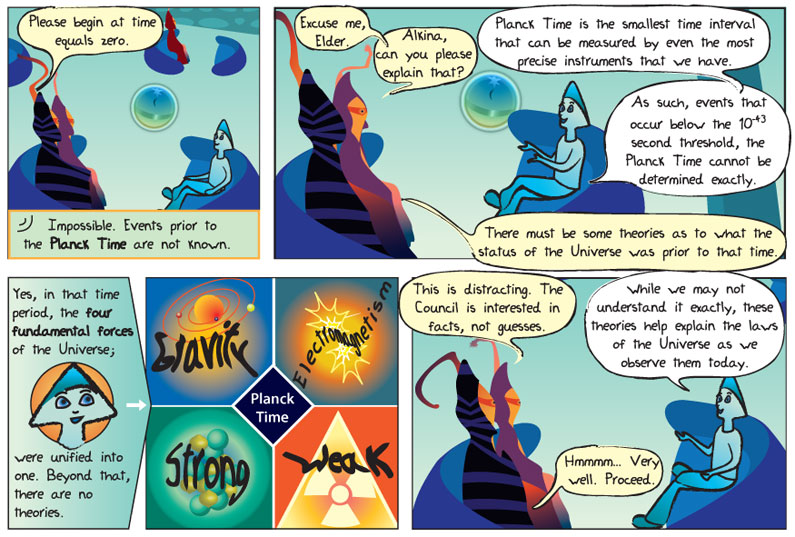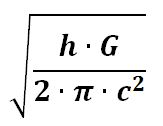## Eposode 87: Planck Time## Transcript:

Panel 1
Worldship: Please begin at time equals zero.
Epo: Impossible. Events prior to the Planck Time are not known.

Panel 2

Worldship: Excuse me, Elder. Alkina, can you please explain that?
Alkina: Planck time is the smallest time interval that can be measured by even the most precise instruments that we have. As such, events that occur below the 10 to the negative 44 threshold, the Planck Time, cannot be determined exactly.
Worldship: There must be some theories as to what the status of the universe was prior to that time.

Panel 3
Alkina: Yes, in that time the four fundamental forces of the universe were unified into one. Beyond that, there are no theories.

Panel 4
Worldship: This is distracting. The council is interested in facts, not guesses.
Alkina: While we may not understand it exactly, these theories help explain the laws of the Universe as we observe them today.
Worldship: Hmmm… very well. Proceed.

## What does it mean?

Planck Time – Planck Time is the unit of time that is determined by unit, or dimensional, analysis using fundamental constants such the speed of light, gravitational constant and the value of (Pi). It is approximately equal to 5.4 x 10-44seconds.

## In human speak please!

Besides being a really small number, there is another reason why time intervals smaller than the Planck Time cannot be measured. The equation below determines how the Planck Time is calculated.Where h is the Planck Constant, G is the gravitational constant and c is the speed of light in a vacuum. The Planck Constant plays a significant role in the uncertainity principle, which says that it is impossible to measure the momentum or the position of a sub-atomic particle with absolute precision. An alternative statement of the principle states that we cannot know both the energy and the time required for a process to arbitrary precision. Newton’s gravitational constant G is always found in gravitational laws, both Newtonian and Einstein’s General Relativity. The speed of light is always found in the laws of special relativity and general relativity and it plays a fundamental role in theories of electricity and magnetism. The presence of Planck’s constant and Newton’s gravitational constant, both small factors, in the numerator, and the speed of light squared, which is a large factor, in the denominator, make the Planck Time very, very small.

The smallest time interval measured thus far is about one zeptosecond, or 1 x 10-21 seconds. This is the same as 0.000000000000000000001 seconds, which is 1023 (1 followed by 26 zeroes) larger than the Planck Time.